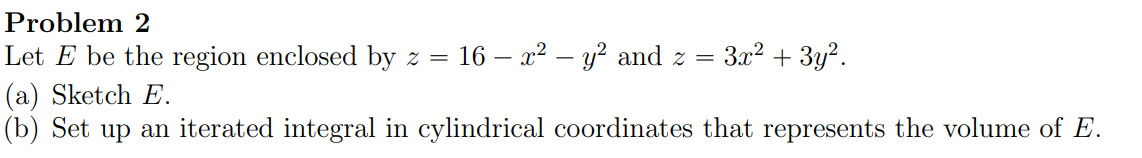# (Solved): Problem 2 Let E be the region enclosed by z=16x2y2 and z=3x2+3y2. (a) Sketch E. (b) Set up a ...Problem 2 Let be the region enclosed by and . (a) Sketch . (b) Set up an iterated integral in cylindrical coordinates that represents the volume of .

We have an Answer from Expert